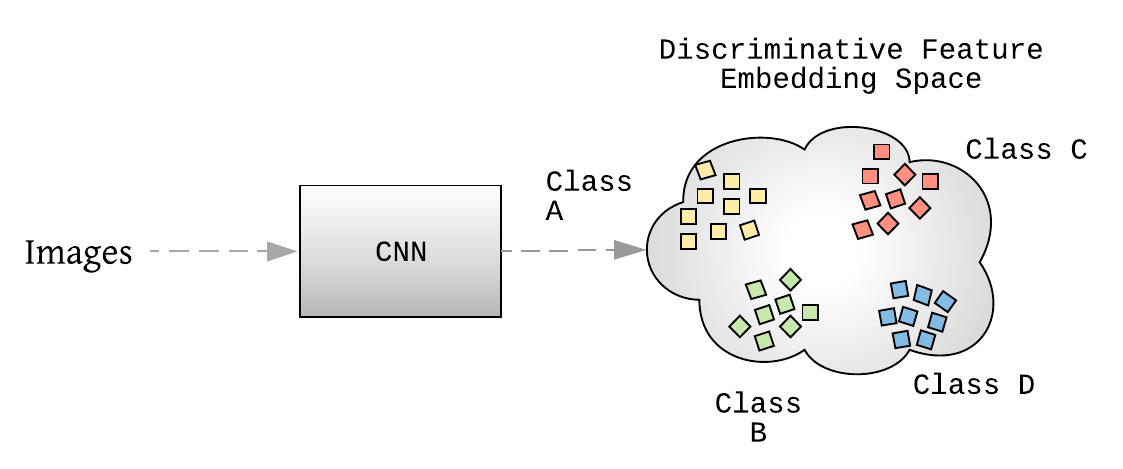# Learning To Differentiate using Deep Metric Learning

Original article was published by Jay Patel on Artificial Intelligence on Medium# Learning To Differentiate using Deep Metric Learning

Recently, machine learning algorithms have contributed greatly to developing very efficient visual search workflows using Convolutional Neural Networks (CNNs). Since data volumes have been increased in recent times, object recognition models are able to recognize the objects and generalize the image features at scale. However, in a challenging classification setting where the number of classes is huge, there are several constraints one needs to address to design effective visual search workflows.

• An increasing number of object categories also increases the number of weights in the penultimate layer of CNN. It makes it hard to deploy them on the device as they also end up increasing the model size.
• When there exist only a few images per class it is difficult to achieve better convergence, making it hard to achieve good performance under various illumination differences, object scale, background, occlusion, etc.
• In an engineering space, it is often required to design visual search workflows that are adaptive to the product ecosystem that contains non-stationary products or it changes as per seasonal trends or geographic locations. Such circumstances make it tricky to train/finetune model in recurring (training after certain time intervals) or online (training for realtime data) fashion.

# Deep Metric Learning

To alleviate these issues, deep learning and metric learning collectively form the concept of Deep Metric Learning(DML), also known as Distance Metric Learning. It proposes to train a CNN based nonlinear feature extraction module (or an encoder), that embeds the extracted image features (also called embeddings) that are semantically similar, onto nearby locations while pushing dissimilar image features apart using an appropriate distance metric e.g. Euclidean or Cosine distance. Together with the discriminative classification algorithms, such as K-nearest neighbor, support vector machines, and Naïve Bayes, we can perform object recognition tasks using extracted image features without being conditioned about the number of classes. Note that, the discriminative power of such a trained CNN module describes features with both the compact intra-class variations and separable inter-class differences. These features are also generalized enough, even for distinguishing new unseen classes. In the following section, we will formalize the procedure to train and evaluate a CNN for DML using a pair based training paradigm.

## Formalism

Let X = {(xᵢ, yᵢ)}, i ∈ [1, 2,… n] be a dataset of n images, where (xᵢ, yᵢ) suggests iᵗʰ image and its corresponding class label. The total number of classes present in the dataset is C, i.e., yᵢ ∈ [1, 2,… C]. Let’s consider f(xᵢ) a feature vector (or an embedding) corresponding to an image xᵢ ∈ Rᴰ, where f: Rᴰ→Rᵈ is a differentiable deep network with parameters θ. Here, D and d refers to the original image dimensions and feature dimensions respectively. Formally we define the Euclidean distance between two image features as Dᵢⱼ = ||f(xᵢ) — f(xⱼ)||², that is the distance between deep features f(xᵢ) and f(xⱼ) that correspond to the images xᵢ and xⱼ respectively. Note that although we are concerned about the Euclidean distance here, in literature there are several other metrics often used to optimize the embedding space. We will discuss this in future posts.

To learn a distance metric function f, the majority of DML algorithms use a relative similarity or absolute similarity constraints using a pair or triplet bases approaches as suggested in Fig. 2 and Fig. 3. A triplet of images can be defined as (f(xᵢ), f(xⱼ), f(xₖ)), where f(xᵢ), f(xⱼ) and f(xₖ) correspond to the feature vectors of an anchor xᵢ, positive xⱼ, and negative image xₖ respectively. xᵢ and xⱼ share similar class labels whereas xₖ has a class different class label than that of the anchor and positive image. A pair of image features corresponding to an image pair(xᵢ,xⱼ), is defined as (f(xᵢ),f(xⱼ)). It refers to as a positive pair if both the images share similar labels, and negative pair otherwise.
The whole procedure of training the end-to-end DML model can be summarized as shown in Fig. 4. Initially, to get the idea of cluster inhomogeneity a batch of images is sampled. Each batch contains object classes P with Q images each class. We use this batch to form one or more mini-batches using a sampling strategy discussed below. These mini-batches are used to compute loss and perform training via backpropagation. Let’s summarize the training procedure to train a deep learning model using DML loss functions. Later, we will discuss a few important training components of this framework, sampling, and loss function.